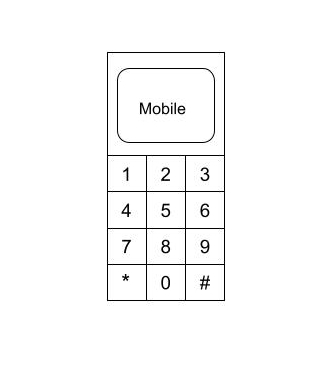# Mike and Mobile

Posted: 8 Jan, 2021
Difficulty: Moderate

## PROBLEM STATEMENT

#### Mike is a little boy who loves solving math problems. One day he was playing with his mom’s mobile. The mobile keypad contains 12 buttons { 10 digits(0-9) and 2 characters(‘*’ and ‘#’) }. Mike wants to know how many different numbers he can generate after pressing exactly the 'N' buttons on the keypad. Mike presses the buttons with the following rules:

``````1. He always presses the button which has a digit written on it, i.e., he never presses the ‘*’ and ‘#’ button.
2. Once he presses a button, the next button he presses should either be the same button or the button which is adjacent to the previous button.
3. In starting he can press any button except ‘*’ and ‘#’.
``````#### Mike is too little to solve this problem. Help Mike to solve this problem. As the answer can be large, so find the answer modulo 10^9 + 7.

##### Input format :
``````The first line of input contains a single integer 'T', representing the number of test cases or queries to be run.

Then the 'T' test cases follow.

The first and only line of each test case contains a positive integer N, which represents the number of buttons to press.
``````
##### Output Format :
``````For each test case, print the number of numbers that can be generated after pressing exactly the N buttons on the keypad.

The output of each test case is printed in a separate line.
``````
##### Note:
``````You do not need to print anything, it has already been taken care of. Just implement the given function.
``````
##### Constraints :
``````1 <= T <= 10
1 <= N <= 5 * 10^4

Time Limit: 1 sec
``````Approach 1

We will recursively find the different numbers we can generate after pressing N buttons on the keypad.

For simplicity, we will define the keypad with a 2D matrix.

keypad[][] = {{1, 2, 3}, {4, 5, 6}, {7, 8, 9}, {-1, 0, -1}}

Below is the detailed algorithm:

Make a recursive function ‘generateNumbersHelper’ which will return the number of numbers we can generate after pressing ‘N’ buttons on the ‘KEYPAD’.

1. Call the function: generateNumbersHelper('COUNT', ‘PREV’), where ‘COUNT’ denotes the number of buttons pressed and ‘PREV’ denotes the previous button pressed.
2. If 'COUNT' is equal to ‘N’, return 1.
3. Make a variable ‘ANS’ which stores the count of different numbers we can generate after pressing ('N' - 'COUNT') buttons on the ‘KEYPAD’. Initialise ‘ANS’ with 0.
4. Check every possible button which we can press in the current state.
• Let ‘R’ denote the row of ‘PREV’ in the ‘KEYPAD’ matrix and ‘C’ denote the column of ‘PREV’ in the ‘KEYPAD’ matrix.
• There are at most 5 possibilities, { ('R', 'C'), ('R'-1, 'C'), ('R'+1, 'C'), ('R', 'C'-1), ('R', ‘C’+1)}.
• Let’s say we press the button with index ('X', ‘Y’), then ('X', 'Y') must be inside the ‘KEYPAD’ matrix and ‘KEYPAD[X][Y]’ must not be equal to -1.
• Update the ‘ANS’, ‘ANS’ += generateNumbersHelper('COUNT'+1, 'KEYPAD[X][Y]').
5. Return the ‘ANS’.